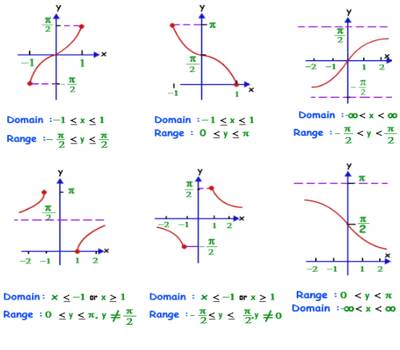Trigon” is Greek for the triangle, and “metric” is Greek for measurement. The trigonometric ratios are special measurements of a right triangle (a triangle with one angle measuring 90°). Remember that the two sides of a right triangle which form the right angle are called the legs, and the third side (opposite the right angle) is called the hypotenuse.

• The sine of the angle is the ratio of the length of the side opposite the angle divided by the length of the hypotenuse. It is the trigonometric function that is equal to the ratio of the side opposite a given angle (in a right-angled triangle) to the hypotenuse.
• The cosine of the angle is the ratio of the length of the side adjacent to the angle divided by the length of the hypotenuse. It is the trigonometric function that is equal to the ratio of the side adjacent to an acute angle (in a right-angled triangle) to the hypotenuse.
• The tangent of the angle is the ratio of the length of the side opposite the angle divided by the length of the side adjacent to the angle.

Restrictions on Trigonometrical Ratios

Some of the Restrictions of Trigonometrical Ratios are:

If A and B two acute angles and sin A = sin B, then we get A = B. If we cancel sign from both sides to get the result, that is wrong.

For Example: If Sin θ = 60°, then θ = 60°. But we should not cancel sign from both sides to get the result.

Cos 2θ ≠ 2 Cos θ

Sin A/Sin B ≠ A/B

Sin A ± Sin B ≠ Sin (A ≠ B)

Cos θ does not imply cos × θ; in fact, it represents the ratio of perpendicular and hypotenuse with respect to the angle θ of a right-angled triangle.

Cos2 θ means (cos θ)2 or cos θ × cos θ; do not write (cos θ)2 = cos θ2 since cos θ2 implies cos (θ × θ).Similarly, we write,

sin3 θ for (sin θ)3;

tan5 θ for (tan θ)5;

sec7 θ for (sec θ)7; etc,

These are the restrictions of trigonometrical ratios need to be followed in case of learning the trigonometric ratios.

Information Source: